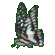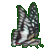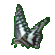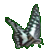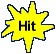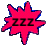Calculus Derivative Rules for Roots     Math Help Fun     Game Tips:

- The derivative power rule is used to get the derivative of a root.

- In general, the derivative power rule for y=xn yields the derivative  dy/dx = nxn-1.
For example, the derivatives of y=x4 and s(t)=t6 are respectively  dy/dx=4x3 and s'(t)=6t5.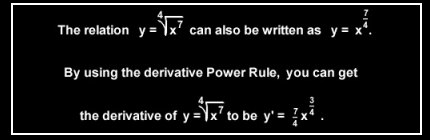- Your Game Score is reduced by the number of butterfly hits.

- To slow the game speed repeat tap/click on the word Slider.
- To increase the game speed repeat tap/click on the word Math.
- Speed can also be adjusted with a keyboard's - and + keys.

- Refresh/Reload the web page to restart the game.